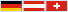Search for Solutions of the n-Queens Problem  (JavaScript required) Aim: n queens are to be placed on a   n x n chessbord such that no queen attacks any other one How the program works:  Input can be made for the width n of the chessboard (4 ≤ n ≤ 30). The first n green colored fields can be filled with at most n different numbers j (1 ≤ j ≤ n). Input of j in column i means: a queen is placed in row j (counted from below) of column i (counted from the left) of the board ("user's input"). Alternatvely, a random distribution of at most n queens on the board can be gererated ("random queens"). Then the lexicographically next position π, giving a solution of the n queens problem (i.e.: the n sums i + π(i) are different and the n differences i - π(i) are different, also), can be computed. ("start/continue"). Empty input leeds to the lexicographically first possible solution. If any input does not produce a solution, the set of prescribed queens can be made smaller by deletion of some numbers, followed by clicking "user's input" again. input n:     (4 ≤ n ≤ 30) state: actually computed position π   (meaning of π:   a queen is placed in row π(i) of the i-th chessboard column.) show the queens on a chessboard: hint: for 25 ≤n, long computing times are possible, a faster application is here. © H. B. Meyer         Eight Queens Problem         deutsche Seite